## Algebra

Showing posts with label sample exam. Show all posts
Showing posts with label sample exam. Show all posts

### Elementary Algebra (Algebra 1) Sample Exams

A complete set of sample exams covering topics found in the first 7 chapters of the online textbook Elementary Algebra.

You will find mobile friendly solutions as well as links to printable copies in pdf format. Please feel free to copy and paste anything you find here into your LMS.

### Intermediate Algebra Exam #3

Click on the 10 question exam covering topics in chapter 6. Give yourself one hour to try all of the problems and then come back and check your answers.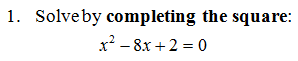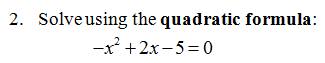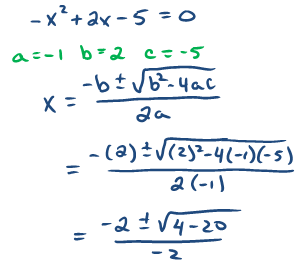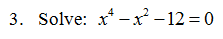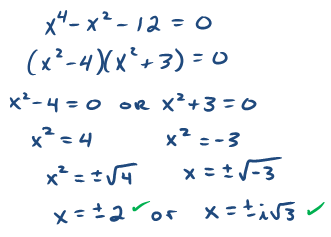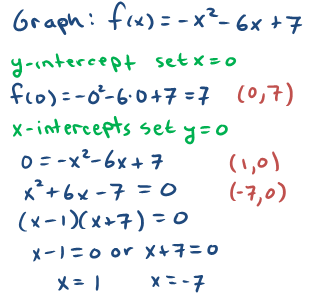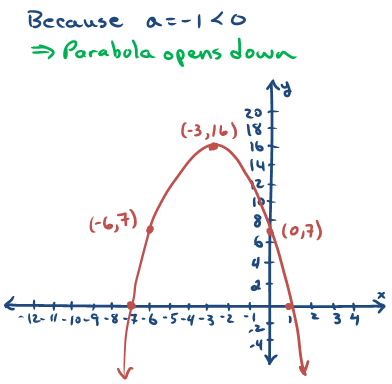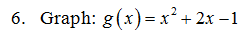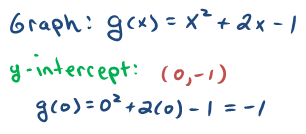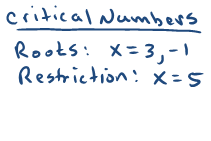9. The height of a baseball in feet tossed upward is given by the functionwhere t represents time in seconds.  What is the maximum height of the baseball?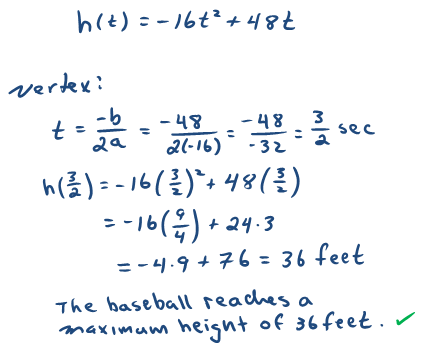10. Find a quadratic equation with solutions: {±5i}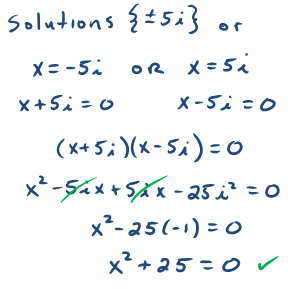---

### Elementary Algebra Exam #1

Click on the 10 question exam covering topics in chapters 1 and 2. Give yourself one hour to try all of the problems and then come back and check your answers.

Simplify: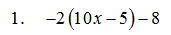Solve: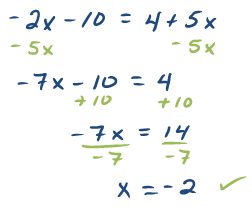Solve and graph all solutions on a number line.10. The perimeter of a rectangle is 54 feet.  If the length is 3 feet less than twice the width, find the dimensions of the rectangle. (Set up an algebraic equation and use it to solve this problem.)